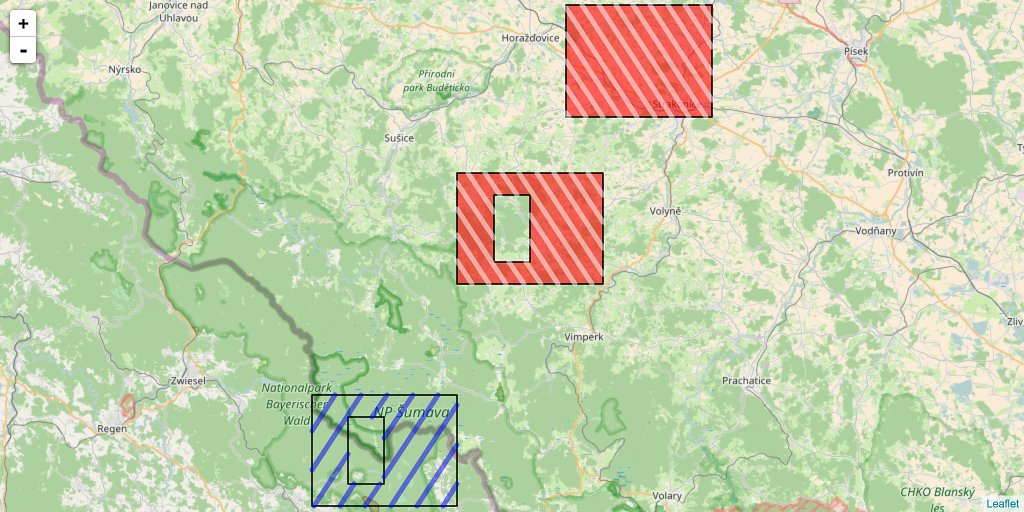# How to fill a hatched area polygon with holes in leaflet with R

## Draw SpatialPolygons with holes using hatched area

Drawing polygons filled with a hatched background is quite easy with classical plot in R. This only requires to define `density` and `angle` parameters of `polygon` function. `SpatialPolygons` from library `sp` also uses this `polygon` function. However, if you want to draw a hatched area of a `SpatialPolygons` when there are holes, this may not work perfectly as the hole is filled with the plot background color to hide the hatched area of the surrounding polygon. Hence, if you want to draw a polygon with holes over a background image, you will not be able to see what is behind holes, which is a shame because this is one aim of a hole. Here, I show how you could get rid of this behaviour by using `SpatialLines` to draw the hatched area. By the way, this trick is also useful for polygons in leaflet widgets as, to my knowledge, hatched polygons is not implemented. Some may want to draw hatched areas instead of coloured polygons with transparency, in particular when there is area superimposition.

### A library on github

I built a R package, available on my statnmap github, to provide the function `hatched.SpatialPolygons`. This function is based on the plot method for SpatialPolygons of library sp. I modified the functions and sub-functions to remove all code for direct drawing and allow to output a SpatialLinesDataFrame object, which can then be drawn over any plot, with or without the original SpatialPolygons. A vignette is provided with the library, you can get the complete Rmarkdown code of the present article here.

``````devtools::install_github("statnmap/HatchedPolygons", build_vignettes = TRUE)
library(HatchedPolygons)

### Draw hatched areas in polygons

Let’s create two polygons, one representing a hole inside the other and draw it with classical `polygon` function.

``````library(dplyr)
library(sp)
library(sf)
library(raster)
library(HatchedPolygons)

# Create two polygons: second would be a hole inside the first
xy = cbind(
x = c(13.4, 13.4, 13.6, 13.6, 13.4),
y = c(48.9, 49, 49, 48.9, 48.9)
)
hole.xy <- cbind(
x = c(13.5, 13.5, 13.45, 13.45, 13.5),
y = c(48.98, 48.92, 48.92, 48.98, 48.98)
)

plot(xy)
polygon(xy, density = 20)
polygon(hole.xy, density = 20, angle = -45, col = "blue")``````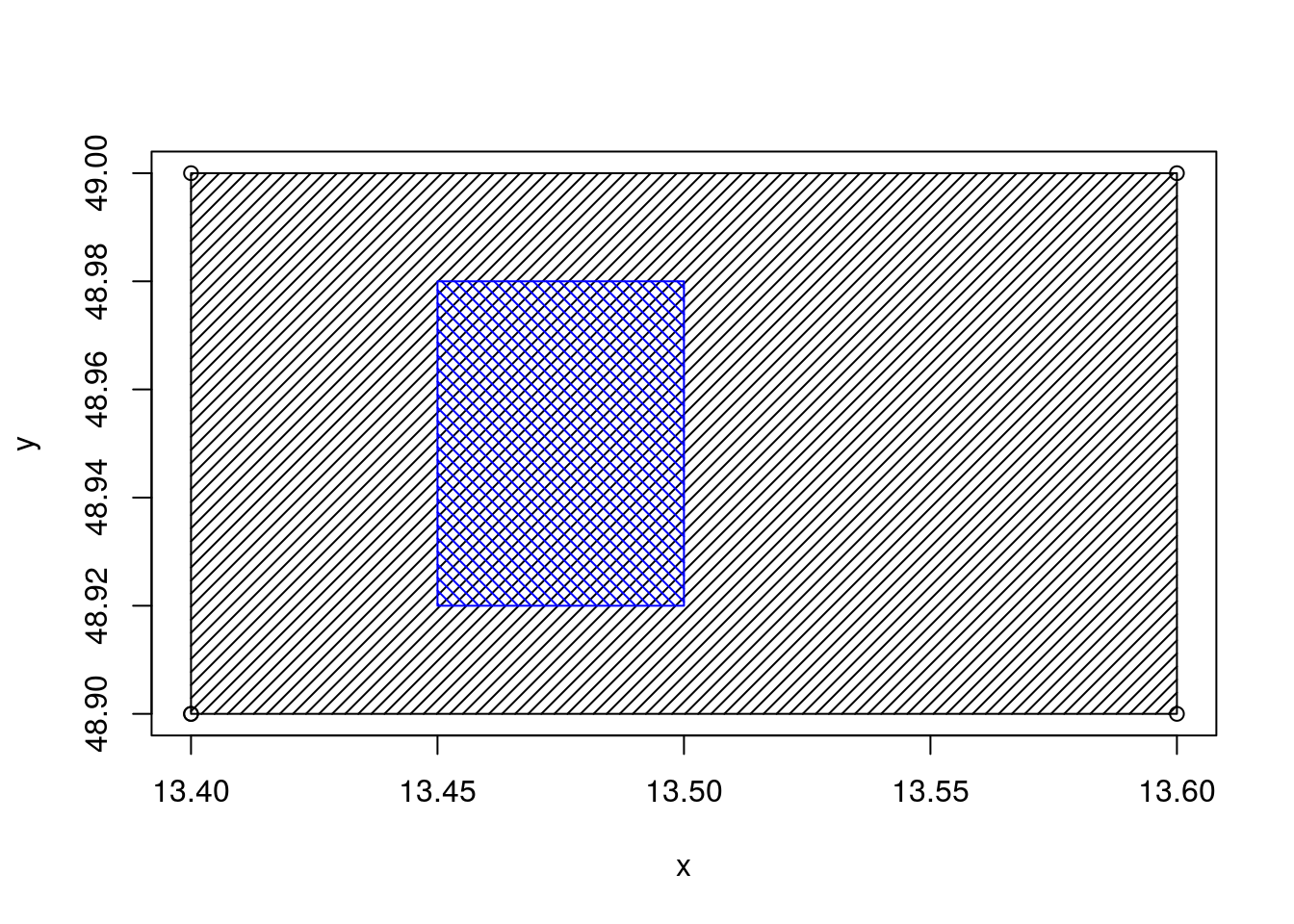### Draw hatched areas in multiple SpatialPolygons

Let’s duplicate polygons at different positions and transform these polygons as `SpatialPolygons`, including holes. Using default graphical options, polygons can be plotted with hatched areas and holes are visible. Polygon drawing function uses the color of the background to fill holes, so that they appear as hole. However, if you want to superimpose your layer over another layer, holes will hide the background image.

``````# Transform as SpatialPolygon to plot
xy.sp <- SpatialPolygons(list(
Polygons(list(Polygon(xy),
Polygon(hole.xy, hole = TRUE)), "1"),
Polygons(list(Polygon(xy + 0.2),
Polygon(xy + 0.35),
Polygon(hole.xy + 0.2, hole = TRUE)), "2")
))

par(bg = "lightblue") # default
plot(xy.sp, density = 20, col = 2:3)``````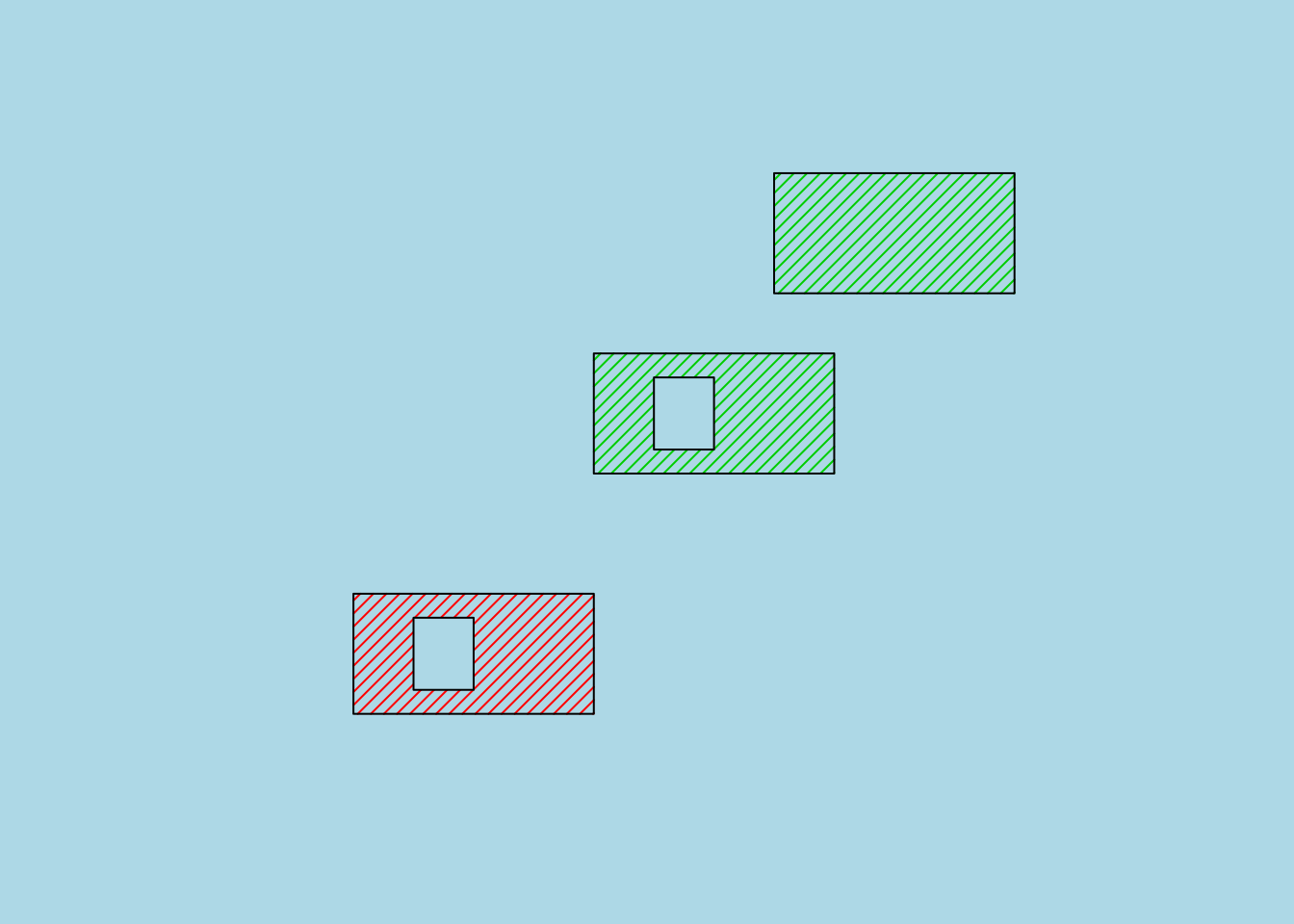``````# Let's define a raster to be used as background
r <- raster(nrows = 50, ncols = 50)
extent(r) <- extent(xy.sp)
r <- setValues(r, 1:ncell(r))

# Draw again polygons with holes
par(bg = "lightblue")
plot(r, legend = FALSE)
plot(xy.sp, density = 20, col = 2:3, add = TRUE)``````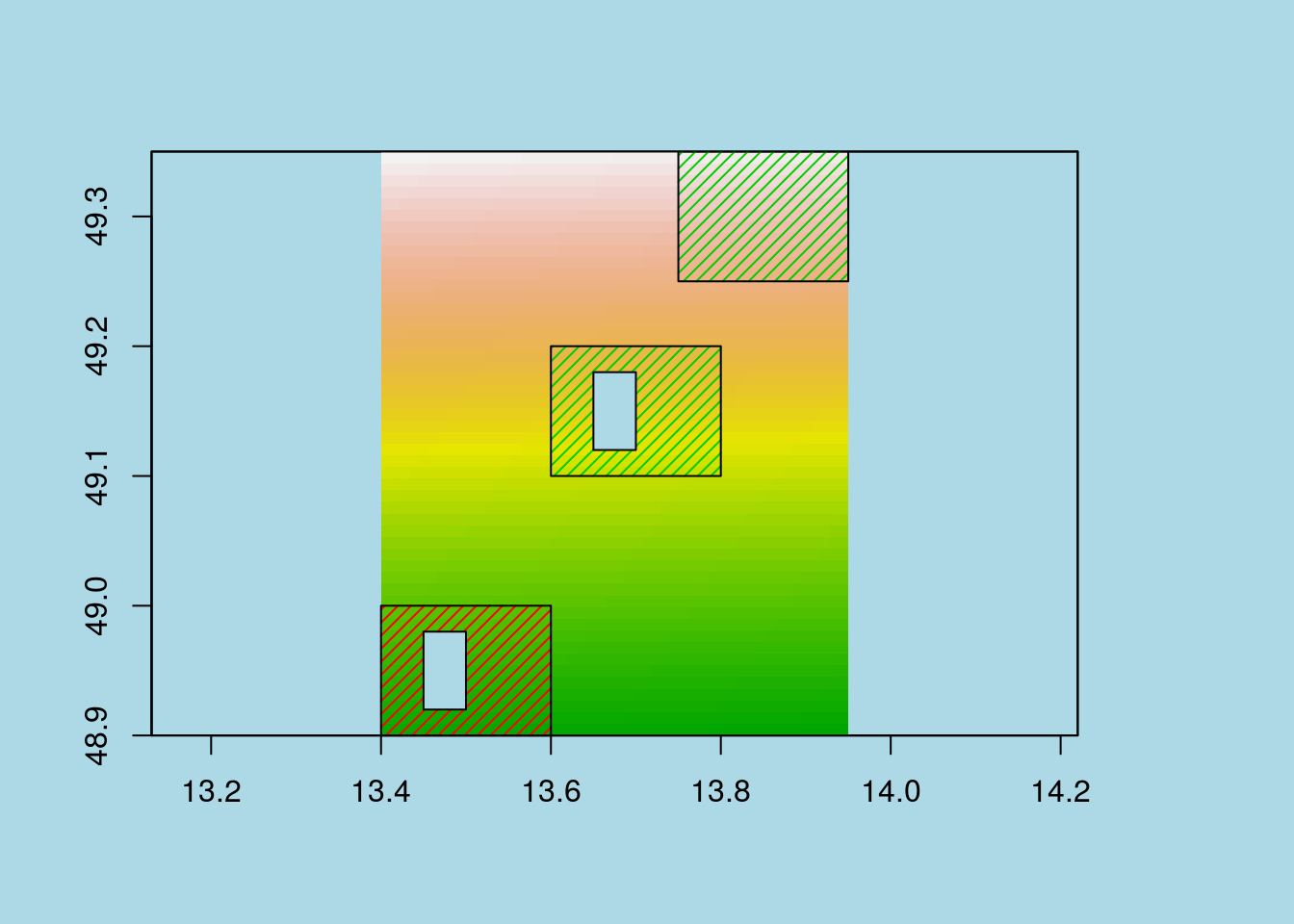### Create SpatialLines to draw SpatialPolygons hatched areas, out of the holes

To avoid filling holes with hatched lines, I decided to use SpatialLines and crop lines that were over a hole using library rgeos. I had to account for multiple polygons and thus created a dataframe with the SpatialLines to record original polygons ID. Thus, the number of features in the SpatialLines is not the same than the original SpatialPolygons but the ID column should allow to retrieve the correct polygon and define common colors for instance.

``````# Allows for density different for each polygon
xy.sp.hatch <- hatched.SpatialPolygons(xy.sp, density = c(60, 90), angle = c(45, 135))

# Draw again polygons with holes
par(bg = "lightblue")
plot(r, legend = FALSE)
plot(xy.sp, col = c("blue", "red"), add = TRUE)
plot(xy.sp.hatch, col = c("cyan", "grey40")[as.numeric(xy.sp.hatch\$ID)], lwd = 2, add = TRUE)``````### Draw hatched SpatialPolygons in leaflet

An interesting possibility of the function is that it can also be used for leaflet widgets, which, to my knowledge, lacks the possibility to fill polygons with a hatched area. (Be careful when using colornames with leaflet, they should exist in css/html, otherwise, you should use hexa code)

``````library(leaflet)

m <- leaflet() %>%
urlTemplate = "https://{s}.tile.openstreetmap.org/{z}/{x}/{y}.png") %>%
fillColor = c("transparent", "red"),
color = "#000000",
opacity = 1,
fillOpacity = 0.6,
stroke = TRUE,
weight = 1.5
) %>%
color = c("blue", "#545454")[as.numeric(xy.sp.hatch\$ID)])``````

### Draw hatched SpatialPolygons in ggplot2

Of course, this can also be transformed with library `sf` to be used with `ggplot2` (specific case for multiple polygons with holes in ggplot on stackoverflow).

``````library(ggplot2)

xy.sp.hatch.sf <- sf::st_as_sf(xy.sp.hatch)
xy.sp.sf <- sf::st_as_sf(xy.sp) %>%
mutate(ID = factor(1:2))

ggplot() +
geom_sf(data = xy.sp.sf, aes(fill = ID)) +
geom_sf(data = xy.sp.hatch.sf, aes(colour = ID)) +
scale_colour_manual(values = c("#6BA5F7", "#545454"))``````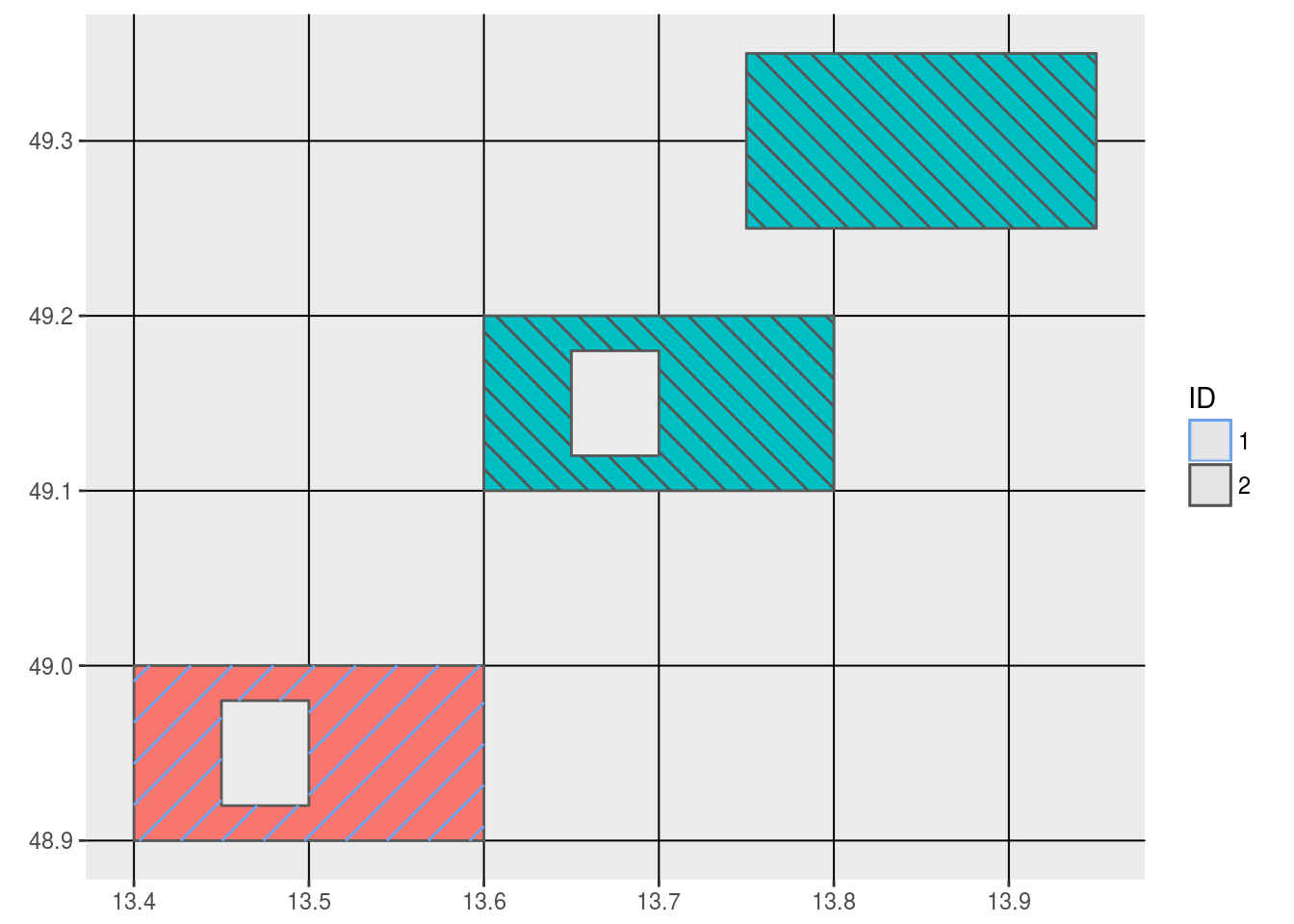Function `hatched.SpatialPolygons` can be found in my R package `HatchedPolygons` on my statnmap github. This R package has been built only for this function

###### Citation:

``` Rochette Sébastien. (2017, May. 23). "How to fill a hatched area polygon with holes in leaflet with R". Retrieved from https://statnmap.com/2017-05-23-how-to-fill-a-hatched-area-polygon-with-holes-in-leaflet-with-r/. ```
``````@misc{Roche2017Howto,